# intersection graph

## Straight line representation of planar linear hypergraphs ★★

Author(s): de Fraysseix; Ossona de Mendez

Conjecture   Every planar linear hypergraph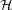has a straight line representation in the plane which maps each vertexto a point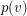and each edge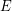to a straight line segment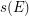, in such a way that:
\item for each vertexand each edge, we have:\item for each couple of distinct edges, we have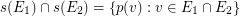Keywords: intersection graph; planar hypergraph